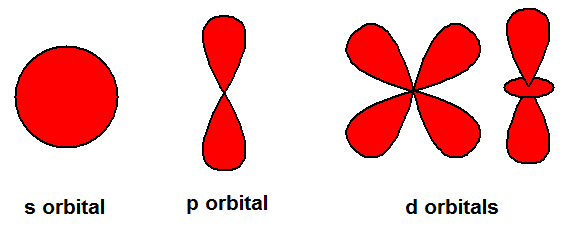# Problem: How do the 2s and 3p orbitals differ from the 1s and 2p orbitals?Match the words in the left column to the appropriate blanks in the sentences on the right.the same shape asa different shape thanlarger insmaller inthe samemorelessthe same amount ofThe 2s orbital would be ________ the 1s orbital but would be ________ size, and the 3p orbitals would have ________the 2p orbitals but would be ________ size. Also, the 2s and 3p orbitals would have ________ nodes.

###### FREE Expert Solution

To determine how the 2s and 3p orbitals differ from the 1s and 2p orbitals:

Recall shapes of the s, p, and d orbitals.• principal quantum number, n size and energy of orbital
• ↑n bigger size, higher energy of orbital  ↑# of nodes (n-1)
83% (292 ratings)###### Problem Details

How do the 2s and 3p orbitals differ from the 1s and 2p orbitals?

Match the words in the left column to the appropriate blanks in the sentences on the right.

the same shape as

a different shape than

larger in

smaller in

the same

more

less

the same amount of

The 2s orbital would be ________ the 1s orbital but would be ________ size, and the 3p orbitals would have ________

the 2p orbitals but would be ________ size. Also, the 2s and 3p orbitals would have ________ nodes.

Frequently Asked Questions

What scientific concept do you need to know in order to solve this problem?

Our tutors have indicated that to solve this problem you will need to apply the Quantum Numbers: Nodes concept. You can view video lessons to learn Quantum Numbers: Nodes. Or if you need more Quantum Numbers: Nodes practice, you can also practice Quantum Numbers: Nodes practice problems.

What professor is this problem relevant for?

Based on our data, we think this problem is relevant for Professor Rines' class at PBCC.